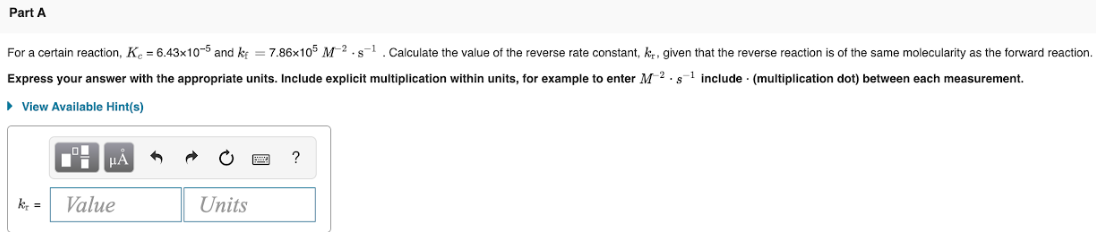# For a certain reaction, Kc = 6.43x10^-5 and kf = 7.86x10^5 M^-2 • s^-1 . Calculate the value of the reverse rate constant. kr, given that the reverse reaction is of the same molecularity as the forward reaction. Express your answer with the appropriate units. Include explicit multiplication within units, for example to enter M^-2 . s^-1 include. (multiplication dot) between each measurement.